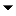﻿ Operations > Trade Performance > Statistics Definitions

Statistics Definitions

 Navigation:  Operations > Trade Performance > Statistics DefinitionsShow/Hide Hidden Text

The following are definitions and formulas used for Trade Performance statistics.

 Notes:   •Quantity is defined as the number of contracts traded•Point value is defined as the monetary conversion of each point (e.g. 100 for currency pairs)•FX lot size is the default Forex Lot Size for the account•Please also review the information on Profit and Loss Calculation Modes where noted as applicableUnderstanding ProfitUnderstanding Total Net ProfitUnderstanding Gross ProfitUnderstanding Gross LossUnderstanding CommissionUnderstanding Profit FactorUnderstanding Max. DrawdownUnderstanding Sharpe RatioUnderstanding Sortino RatioUnderstanding Ulcer IndexUnderstanding ProbabilityUnderstanding Winning, Losing, Even, and Total Number of TradesUnderstanding Average TradeUnderstanding Average Winning TradeUnderstanding Average Losing TradeUnderstanding Ratio Avg Win / Avg LossUnderstanding Maximum Consecutive WinnersUnderstanding Maximum Consecutive LosersUnderstanding Largest Winning TradeUnderstanding Largest Losing TradeUnderstanding Average # of trades per dayUnderstanding Average Time in MarketUnderstanding Profit Per MonthUnderstanding Max. Time to RecoverUnderstanding Longest Flat PeriodUnderstanding Average MAEUnderstanding Average MFEUnderstanding Average ETDUnderstanding Cumulative ProfitUnderstanding Entry, Exit, and Total Efficiency